Chapter 12 Electricity Class 10 Science Important Questions and Answers PDF will help you in scoring more marks. This consists of 1 mark Questions, 3 Mark Numericals Questions, 5 Marks Numerical Questions and previous year questions from Chemical Reactions and Equations Chapter.

## Electricity Class 10 Important Questions and Answers Science Chapter 12

Based on Electric Potential and Electric Current

Question 1.
Define electric potential.
Electric potential at a point in an electric field is defined as the work done in moving a unit positive charge from infinity to that point in the electric field.

More Resources

Question 2.
State the relation between work (W), change (q) and electric potential (V).
V = W/q.

Question 3.
What is the S.I. unit of electrical potential ? (CBSE 2005, 2012)
volt.

Question 4.
Define 1 volt electric potential.
Electric potential is said to be 1 volt if 1 Joule of work is done in moving 1 coulomb charge from infinity to a point in the electric field.

Question 5.
Is electric potential a scalar or a vector physical quantity ?
Electric potential is a scalar physical quantity.

Question 6.
What is meant by potential difference between two points ? (CBSE 2011)
Work done per unit charge in moving a unit positive charge from one point to another point in an electric field is called potential difference between two points.

Question 7.
Name the instrument used to measure the electric potential difference. (CBSE 2011, 2012)
Voltmeter.

Question 8.
Write down the relation between the potential difference between two points A and B in a conductor, work done W in moving a unit charge from point B to A and the charge q.
Or
State the relation between work, charge and potential difference for an electric circuit.
[CBSE (All India) 2009, 2013]
Or
Express work done in an electric field in terms of charge and potential difference. (CBSE 2014)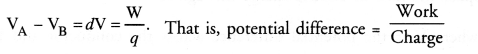Question 9.
Mention the factor that maintains the flow of charge through a conductor. (CBSE 2013, 2014)
Potential difference across the ends of the conductor.

Question 10.
Define electric current.
Electric current is defined as the amount of electric charge flowing through any cross-section of a conductor per unit time.

Question 11.
Write down the relation between the electric current I passing in a conductor, change Q flowing in the conductor and time t.
Or
Write the relation between coulomb and ampere. (CBSE 2014)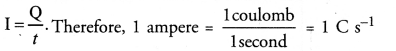Question 12.
State the relation between the current I passing in a conductor, number of electrons (n) flowing through any cross-section of the conductor, magnitude of charge on an electron (e) and time t.
I = ne/t.

Question 13.
State SI unit of electric current.
ampere (A)

Question 14.
Define 1 ampere electric current.
Electric current through a conductor is said to be 1 ampere if 1 coulomb electric charge flows through a cross-section of a conductor in 1 second.

Question 15.
How is the direction of electric current related to the direction of flow of electrons in a wire ?
[CBSE (All India) 2009]
The direction of electric current in a wire is just opposite to the direction of flow of electrons in the wire.

Question 16.
Name the instrument used to measure electric current in a circuit. [CBSE (Delhi) 2008, 2011, 2012, 2015]
Ammeter.

Question 17.
How is ammeter connected in the circuit to measure electric current ? (CBSE 2011, 2012)
Ammeter is connected in series in an electric circuit.

Question 18.
How is voltmeter connected in the circuit to measure electric potential difference ?
Voltmeter is connected in parallel across a conductor or resistor in the electric circuit.

Question 19.
Define ohm’s law. [CBSE 2010, 2011, 2012, 2013, 2015]
Or
State the law that gives the relationship between the potential difference (V) across the two ends of a conductor and the current (I) flowing through it. (CBSE 2012)
Ohm’s law states that the electric current flowing through a conductor is directly proportional to the potential difference across the ends of the conductor, provided the temperature and . other physical conditions of the conductor remain the same.

Question 20.
Graph is plotted between the values of potential difference (V) and current (I). What v conclusion do you draw about the relation between V and I from this graph. State this relation in your words. (CBSE 2013)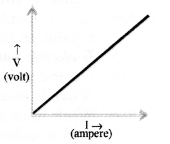The potential difference (V) is directly proportional to the current (I).

Question 21.
Draw a circuit diagram to establish a relationship between potential difference across the ends of a resistor and current flowing through it. (CBSE 2013)Question 22.
State the formula showing how the current I in a conductor varies with the potential difference V applied across it. [CBSE (Delhi) 2004(S), 2012]
V ∝ I or V = IR or I = V/R .

Question 23.
Define electrical resistance of a conductor. (CBSE 2013)
It is the property of a conductor to oppose the flow of electric charge through it.
Resistance of a conductor, R = V/I , where V is the potential difference across the conductor and I is the current flowing through the conductor.

Question 24.
State SI unit of resistance. [CBSE (Delhi) 2004(S), 2012]
ohm (Ω).

Question 25.
“The resistance of a conductor is IΩ”. What is meant by this statement ? (CBSE 2010, 2011, 2012)
Or
Define 1 ohm resistance. (CBSE 2014)
The resistance of a conductor is said to be 1Ω if a potential difference of IV across the ends of the conductor makes a current of 1A to flow through it.

Question 26.
What is the shape of the graph obtained by plotting potential difference applied across a conductor against the current flowing through it ?
A straight line passing through the origin and has constant slope.

Question 27.
What is the name the physical quantity which is equal to V/I ?
Or
Name the physical quantity whose unit is volt-ampere.
y = R. Therefore, physical quantity is resistance of a conductor.

Question 28.
What does the slope of V-I graph at any point represent ?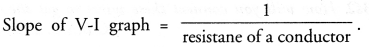Therefore, slope of V-I graph represents reciprocal of the resistance of a conductor resistance of a conductor.

Question 29.
What happens to resistance of a conductor when temperature is increased ?
Resistance of a conductor increases with the increase in temperature.

Question 30.
The voltage — current (V-I) graph of a metallic circuit at two different
temperature T1 and T2 is shown. Which of the two temperatures is higher and why ? (CBSE 2010)
Or
The voltage-current (V-I) graph of a metallic conductor at two different temperatures T1 and T2 is shown in figure. At which temperature is the resistance higher ? (CBSE 2011, 2012)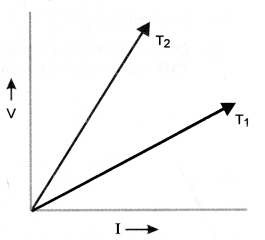Slope of I-V graph = resistance of metallic conductor.
Since, slope of I-V graph at temperature T2 is greater than the slope of I-V graph at temperature T1, therefore, resistance at T2 is greater than resistance at T1 . Since, resistance of a metallic conductor increases with increase in temperature, therefore, T2 > T1.

Question 31.
How does the resistance of a wire vary with its cross-section area ?
Resistance of a wire is inversely proportional to its cross-sectional area. More is cross-sectional area of a conductor, less is the resistance of the conductor.

Question 32.
State the relation between the resistance R of a conductor, resistivity ρ of a conductor, length l of a conductor and area of cross-section A of the conductor.
Or
Write an expression for the resistivity of a substance. (CBSE 2015)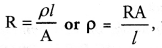where R is the resistance, A is the area of cross-section and l is the length of the substance.

Question 33.
What is electrical resistivity ? [CBSE 2010, 2014, 2015]
Electrical resistivity of a material is defined as the resistance of an object (made of the material) of unit length and unit area of cross-section.

Question 34.
State SI unit of resistivity. [CBSE (Delhi) 2004 (S), 2014, 2015]
ohm-metre (Ω-m).

Question 35.
A wire of resistivity ‘ρ’ is pulled to double its length. What will be its new resistivity ? (CBSE 2010, 2011)
New resistivity will also be ‘ρ’ because resistivity of a wire does not depend on its length.

Question 36.
You have a metal, insulator and an alloy. Write these substances in the ascending order of their electrical resistivity.
Resistivity of metal < resistivity of alloy < resistivity of insulator.

Based on resistors connected in series and parallel

Question 37.
n resistance each of R are connected in series in a circuit. What is the total effective resistance of the circuit ?
(CBSE 2010)
Reff = R1 + R2 + R3 + … upto n = nR

Question 38.
n resistors each of resistance R are connected in parallel in an electric circuit. What is the total effective resistance of the circuit ? (CBSE 2010 )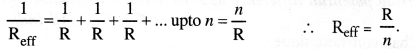Question 39.
n resistors each of resistance R are first connected in series and then in parallel. What is the ratio of the total effective resistance of the circuit in series combination and parallel combination ? (CBSE 2013)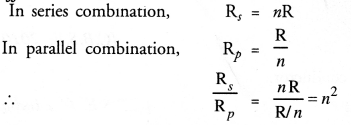Question 40.
You have two metallic wires of resistances 60 and 30. How will you connect these wires to get the effective resistance of 20 ? (CBSE 2010, 2012)
In parallel.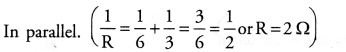Question 41.
Draw a schematic diagram of a circuit consisting of battery of two cells each of 1.5 V, 50 resistor, 100 resistor and 150 resistor and a plug key, all connected in series. [CBSE (All India) 2009, 2011, 2013]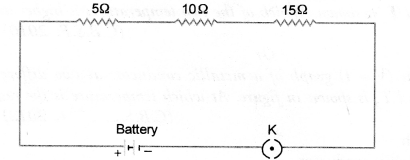Based on heating effect of electric current

Question 42.
What is heating effect of electric current ?
The production of heat in a conductor due to the flow of electric current through it is called heating effect of electric current.

Question 43.
Write down the relation between heat produced H in a conductor of resistance R through which current I passes for t seconds.
H = I2Rr.

Question 44.
State Joule’s law of heating. (CBSE 2012, 2012, 2013, 2014, 2015)
According to Joule’s law of heating, the amount of heat produced in a conductor is

1. directly proportional to the square of electric current passing through the conductor,
2. directly proportional to the resistance of the conductor, and
3. directly proportional to the time for which electric current passes through the conductor.

Question 45.
Write a mathematical expression for Joule’s law of heating. Name one device which works on this principle.
(CBSE 2010, 2015)
H = I2 Rt. An electric heater and electric bulb work on Joule’s law of heating.

Question 46.
What is meant by the statement that the rating of fuse in a circuit is 5A ?
It means maximum current of 5A can pass through the fuse without melting it.

Question 47.
Name the material used for making the filament of a bulb.
Tungsten.
on electric energy and electric power

Question 48.
Define electric energy. (CBSE 2013, 2015)
It is defined as the work done by a source of electricity to maintain electric current in an electrical circuit.

Question 49.
Define electric power.
It is defined as the amount of electric energy consumed in an electric circuit per unit time.

Question 50.
Name the physical quantity expressed as the product of potential difference and electric current.
Electric power.

Question 51.
State SI unit of electric power.
Volt x ampere (or Watt).

Question 52.
Name the unit used in selling electrical energy to consumers.
Or
What is the commercial unit of energy ?
Kilowatt hour (kWh).

Question 53.
Which one is having lesser resistance : A 60 W bulb or a 40 W bulb ?Hence, bulb of higher wattage will have less resistance. In other words, resistance of 60W bulb is less than the resistance of 40 W bulb.

Question 54.
What is the difference between kilowatt and kilowatt hour. (CBSE 2012, 2014)
Kilowatt is the unit of electric power and kilowatt hour is the commercial unit of electric energy.

Short Answer Questions (2 & 3 Marks)

Based on electric current, ohm’s law and resistance

Question 1.
Define electric current. State and define its SI unit. (CBSE 2012, 2013, 2014, 2015)
Electric current is defined as the amount of charge flowing through a cross-section of a conductor in unit time.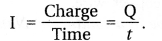SI unit of electric current is ampere (A).
Electric current through a conductor is said to be 1 ampere if one coulomb charge flows through a cross-section of the conductor in one second.

Question 2.
List two differences between a voltmeter and ammeter. (CBSE 2013)

 Ammeter Voltmeter 1.    Ammeter measures electric current in the circuit. 2.     Ammeter is connected in series in an electric circuit. 1.     Voltmeter measures the potential difference be­tween two points on a conductor. 2.     Voltmeter is connected in parallel across the ends of a conductor or resistor.

Question 3.
What is an electric circuit ? Distinguish between an open and a closed circuit.
[CBSE (All India) 2009, 2010, 2011]
electric circuit:
An electric circuit is a closed conducting path containing a source of electric energy (i.e., a cell or a battery) and a device or element or load (say, an electric bulb) utilizing the electric energy.
The direction of electric current is opposite to the direction of the flow of electrons in the conductor.
Open electric circuit : An electric circuit through which no electric current flows is known as open electric circuit.
The electric circuit shown in figure 10 (A) will be open circuit if the plug of the key is taken out or if the connecting wire breaks from any point.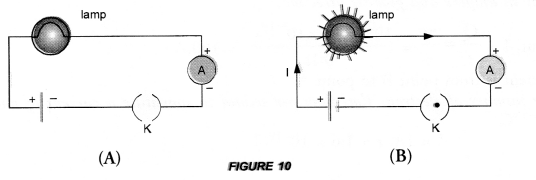Closed circuit : An electric circuit through which electric current flows continuously is known as closed circuit (Figure 10 (B)).

Question 4.
What do the following symbols represent in a circuit ? Write the name and one function of each.
(CBSE 2013)(i) It represents a battery. It maintains a potential difference across the circuit element for the flow of current in the circuit.
(ii) It represents an ammeter. Ammeter is used to measure the electric current in the circuit.

Question 5.
What do the following symbols represent in a circuit ? Write the name and one function of each ?
[CBSE 2011, 2012, 2013, 2015]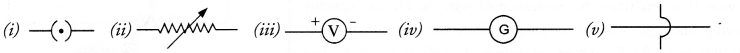(i) It represents a closed plug key. It is used to make the closed electric circuit.
(ii) It represents a variable resistance. It is used to increase or decrease the electric current in the circuit.
(iii) It represents a voltmeter. It is used to measure the potential difference across a resistor in the circuit.
(iv) It represents a galvanometer. It is used to detect the presence of small current in the circuit.
(v) It represents wire crossing (not connected with each other). The wires are used to connect various components in the circuit.

Question 6.
Express Ohm’s law both by a mathematical formula and by a graph line. (CBSE (Delhi) 2004 (S))
Mathematical formula of Ohm’s law is
V = IR
For a graph line,Question 7.
List the factors on which the resistance of a conductor depends. Write the expression to show the relation of resistance with these factors. (CBSE 2013, 2014)
Resistance of a factor depends on its length (l) and area of cross section (A).

Question 8.
What is likely to happen and how it would affect the value of resistance if we pass the current for a longer time ? (CBSE 2014)
When current passes through a conductor for a longer time, the conductor is heated due to Joule’s heating effect. Resistance of conductor increases with increase in temperature due to heating effect.

Question 9.
Two wires, one of copper and other of manganin, have equal lengths and equal resistances. Which wire is thicker ? Given that resistivity of copper is lower than that of manganin. (CBSE 2011, 2015)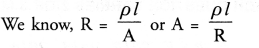Since l and R of both the wires are same, so A p . Since, resistivity ip) of manganin is higher than that of copper, so manganin wire is thicker than that of copper wire.

Question 10.
Mention the condition under which charge can move in a conductor. Name the device which is used to maintain this condition in an electric circuit. (CBSE 2012, 2013)
The ends of the conductor must be maintained at different voltages. This condition is maintained in a conductor by a cell or a battery.

Question 11.
In the circuit diagram shown, the two resistance wires A and B are of the same length and same material, but A is thicker than B. Which ammeter Af or A2 will indicate higher reading for current ? Give reason.
(CBSE 2010, 2011)The current will be high if resistence is low.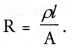p and l for both wires A and B are same but area of cross-section (A) of wire A is- more than the wire B. Therefore, resistance of wire A is less than the resistance of the wire B. Hence, ammeter A connected in series with the wire A will indicate higher reading for current.

Question 12.
In the circuit diagram shown, the two resistance wires A and B are of same area of cross-section and same material, but A is longer than B. Which ammeter A1 and A2 will indicate higher reading of current ? Give reason. (CBSE 2010, 2011)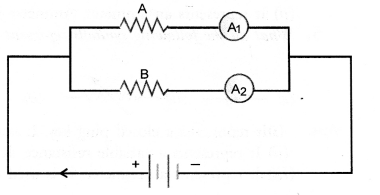The current in the circuit is high if the resistance of the circuit is low. Since wire A is longer than B, so the resistance of wire B is less than the resistance of wire A. Hence, current flowing through wire B is greater than the current flowing through wire A. Therefore, ammeter A2 will indicate higher reading of current.

Question 13.
Two students perform experiments on two given resistors R1 and R2 and plot the following V-I graphs. If R1 > R2, which of the two diagrams correctly represent the situation on the plotted curves ? Justify your answer. (CBSE 2010, 2012)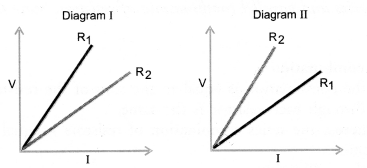Resistance of a conductor = slope of I-V graph. It means, resistance is high if slope of I-V graph for it is steeper. Since R1 > R2, therefore, slope of I-V graph for R1 must be steeper than the slope of I-V graph for R2 Thus, diagram I represents the situation correctly.

Question 14.
Is the circuit given below correct ? Justify your answer. (CBSE 2014)The given circuit is incorrect because in a circuit, ammeter is always connected in series and voltmeter is connected parallel to the resistor R1. The correct circuit is shown in figure.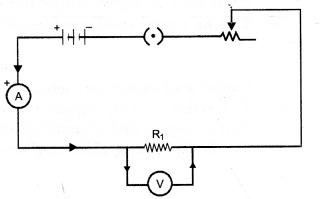Question 15.
List two distinguishing features between the resistance and resistivity of a conductor. (CBSE 2014)

1. Resistance of a conductor depends on the length and area of cross-section of the conductor. On the other hand, resistivity of a conductor is independent of length and area of cross-section of the conductor.
2. Unit of resistance is ohm. Unit of resistivity is ohm-metre.

Based on resistors connected in series and parallel

Question 16.
Draw a schematic diagram of a circuit consisting of a battery of 12V, three resistors of 5Ω, 10Ω and 20Ω connected in parallel, an ammeter to measure the total current through the circuit, voltmeter to measue the potential difference across the combination of resistors. [CBSE (All India) 2008]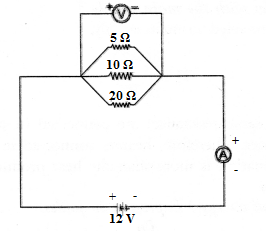Question 17.
(a) Why an ammeter likely to bum out if you connect it in parallel ?
(b) Why is series arrangement not found satisfactory for domestic lights ?(CBSE 2010, 2011)
(a) Ammeter is a low resistance device. If it is connected in parallel, a large current flows through it. Hence, large heat is produced and it may burn the ammeter.
(b) If domestic lights are connected in series, then all lights will be switched off even when only one light fuses.

Question 18.
Draw diagrams to show series and parallel combinations of resistors. State three salient features each of both the combinations. (CBSE 2015)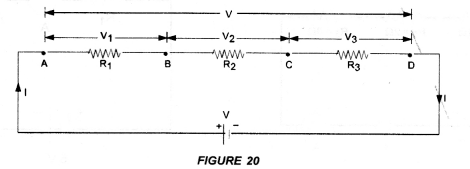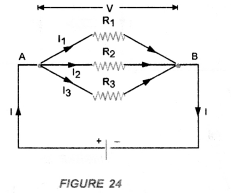Salient features of series combination :

1. The net resistance of the combination is equal to the sum of the resistances of the individual resistor.
2. The current flowing through each resistor is the same.
3. The voltage applied across the series combination of resistors is equal to the sum of potential differences across the individual resistors.

Salient features of parallel combination :

1. The reciprocal of the net resistance of the combination is equal to the sum of the reciprocal of the resistances of the individual resistors.
2. Different amount of current flows through each resistor.
3. The potential difference across each resistor is equal to the voltage applied across the combination.

Based on heating effect of electric current

Question 19.
Why is the tungsten used almost exclusively for filament of electric lamps ?
Or
Why is Tungsten used for the filament in electric bulb ? (CBSE 2011, 2014, 2015)
This is because

1. melting point of tungsten is very high (about 3380°C) and
2. it does not oxidise (or burn) even at higher temperatures.

Question 20.
Why do copper or aluminium wires usually used for electricity transmission and distribution purposes ?
(CBSE 2012, 2013)
Or
Copper and aluminium wires are usually employed for electricity transmission. Explain reason.
The resistivities of copper and aluminium are very low, so electric current flows easily through them. Hence, copper and aluminium wires are usually used for electricity transmission and distribution purpose.

Question 21.
Should the heating element of an electric iron be made of iron, silver or nichrome wire 1
[CBSE (Foreign)2005, 2016]
Or
List two reasons why nichrome is used for making heating element of electrical appliances. (CBSE 2015)
It should be made of nichrome wire because

1. resistivity of nichrome is greater than that of iron and silver,so more heat is produced in the nichrome wire due to the flow of current.
2. melting point of nichrome wire is greater than that of iron and silver and hence it does not melt easily on heating.
3. nichrome wire does not oxidise (or burn) easily even at higher temperature.

Question 22.
Two identical immersion heaters are to be used to heat water in a large container. Which one of the following
arrangement would heat the water faster,

1. connecting the heaters in series with the main supply,
2. connecting the heaters in parallel with the main supply ? (CBSE 2011)

Heat produced in a heater, when connected to main supply,When identical heaters (i.e. having same resistance) are connected in parallel, their net resistance decreases as compared to when connected in series. Therefore, heaters connected in parallel would heat the water faster as the heat produced in parallel combination is more than the heat produced in series combination, on electric energy and electric power

Question 23.
What is commercial unit of electrical energy ? Express it in joules. (CBSE 2015)
Or
Define 1 kWh. How is this unit of energy related to 1 joule ? (CBSE 2011, 2015)
Or
Establish the relationship between 1 kWh and SI unit of energy (joule). (CBSE 2012, 2013, 2015)
Commercial unit of electrical energy is kWh. 1 kWh is the amount of electric energy consumed by 1000 W electric appliance when operates for 1 hour. 1 kWh = 1000 W x 3600 s = 1000 Js-1 x 3600 s = 3.6 x 106 J.

Question 24.
Two electric bulbs A and B are marked 220V, 40W and 220 V, 60W respectively. Which one of the two has greater resistance ? (CBSE 2010, 2011)Therefore, bulb A (40 W) has greater resistance than the bulb B (60 W)

Question 25.
Define electric power. A device of resistance R is connected across a source ofV voltage and draws a current I. Derive an expression for power in terms of voltage and resistance.
Electric power is defined as the amount of electric work or electric energy per unit time.
If W be the amount of electric energy consumed in a circuit in t seconds, then the electric power is given by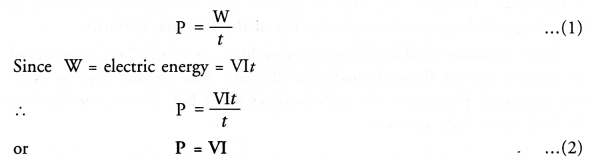Thus, electric power is defined as the product of potential difference applied across the circuit and current flowing through it.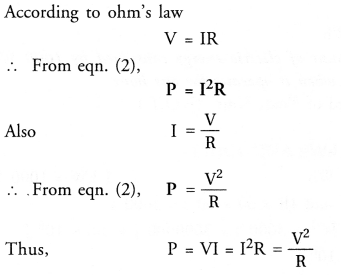Units of Power: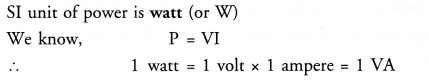Question 1.
State Ohm’s law. How can this law be verified experimentally ? Does Ohm’s law hold good under all conditions.
Comment. (NCERT Question Bank, CBSE, 2010, 2012)
For Ohm’s law: Ohm’s law states that the electric current flowing through a conductor is directly proportional to the potential difference across the ends of the conductor, provided the temperature and . other physical conditions of the conductor remain the same.
For experimental verification: Verify Ohm’s law
Apparatus : A conductor of resistance R, an ammeter, a voltmeter, a battery, a variable resistance (or rheostat used to change the current in the circuit), connecting wires, a key and sand paper.
Procedure:

1. Connect the various components as shown in figure 12.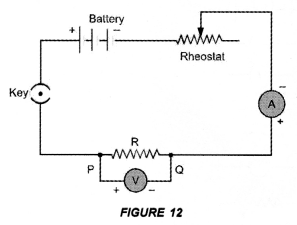2. Close the key, so that current begins to flow in the circuit.
3. Note down the potential difference (V) across the conductor PQ of resistance R shown by the voltmeter and the corresponding current (I) shown by the ammeter.
4. Now move the knob of rheostat so that the current in the circuit increases.
5. Again note down the potential difference (V) across the conductor PQ of resistance R in the voltmeter and current in the circuit shown by ammeter.
6. Repeat the experiment at least five times by increasing the current in the circuit by moving the knob of the rheostat in steps.

Observations:

 S.No Potential Difference  (V) Current (I) V/I 1. 2. 3. 4. 5. 6. 7.

Plot a graph between V and I by taking V along X-axis and I along Y-axis. We get a straight line passing through origin as shown in figure 11.Conclusion: From the graph between V and I, we conclude that I x V, which is Ohm’s law. Hence Ohm’s law is verified experimentally.
Precautions: While verifying Ohm’s law experimentally, the following precautions should be taken :

1. Current should not be allowed to pass through the circuit continuously for a long time, which may cause the increase in temperature of the conductor. Therefore, the plug of the key must be taken out every time after noting the readings of ammeter and voltmeter.
2. Connections should be tight.
3. The conductor used in the experiment should be such that its resistance is not changed with increase in temperature of the conductor.

Ohm’s law holds good if the temperature of the conductor remains the same.

Question 2.
How will you infer with the help of an experiment that same voltage or potential difference exists across three resistors connectred in parallel arrangement to a battery ?
Perform an activity to investigate the relation between potential difference across parallel combination of resistors and the potential difference across each individual resistors,

1. Connect three resistors of resistances R1, R2 and R3 in parallel. One end of each resistor is joined at a common point ‘a’ and the other end of each resistor is connected at another common point ‘b’.
2. Connect the parallel combination of resistors with a battery, a plug key K and an ammeter A as shown in figure 22(A).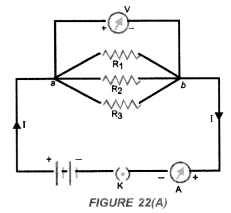3. Now connect a voltmeter across the parallel combination of resistors between a and b points.
4. Note the reading of voltmeter. Let it be V. This is the potential difference across the parallel combination of resistors.
5. Now, disconnect the voltmeter and connect it across R1 as shown in figure 22(B).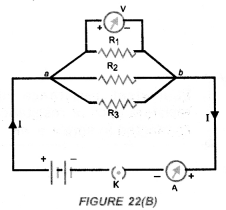6. Note the reading of voltmeter. It is found to be V.
7. Disconnect the voltmeter and connect it across R2. Note the reading of voltmeter. It is found to be V.
8. Again disconnect the voltmeter and connect it across R3. Note the reading of voltmeter. It is found to be V.

Conclusion : When resistors are connected in parallel to each other, potential difference across each resistor is equal to the potential difference across the parallel combination of resistors.

Question 3.
Describe an experiment to study the factors on which the resistance ofa conductor or a conducting wire depends.
For experiment:
Connect the various electrical components as shown in figure 15.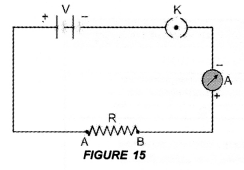1. Dependence of length of a conductor:
Take a copper wire of length l and connect it between the terminals A and B. Note the reading of ammeter. Now take another copper wire of same area of cross-section but of length 2l. Connect it between the terminals A and B by disconnecting the previous wire. Again, note the reading of ammeter. It will be found that the reading of ammeter (i.e., electric current) in the second case is half of the reading of ammeter in the first case. Since R = V/I, so resistance of second wire is double than the resistance of the first wire. Thus, resistance of a conductor is directly proportional to the length of the conductor,
i.e      resistance ∝ length of the conductor
Thus, more is the length of a conductor, more is its resistance.
Thus, the resistance of’a conductor is ‘inversely proportional to the area of cross -section of the conductor.
2. Dependence on area of cross-section of a conductor:
Now take two copper wires of same length but of different area of cross-sections. Let area of cross-section of first wire is more than the area of cross-section of the second wire. Connect first wire between the terminals A and B in the circuit shown in figure 15. Note the reading of ammeter. Now disconnect the first wire and connect the second wire between the terminals A and B. Again note the reading of the ammeter. It will be found that the reading of ammeter (i.e. electric current) is more when first wire (i.e. thick wire) is connected between A and B than the reading of the ammeter when second wire (i.e. thin wire) is connected between the terminals A and B.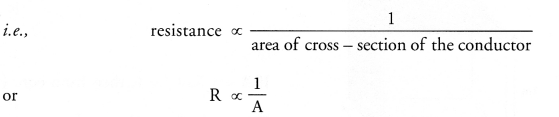Thus, the resistance of a thin wire is more than the resistance of a thick wire.
3. Effect of the Nature of material:
Take two identical wires, one of copper and other of aluminium. Connect the copper wire between the terminals A and B. Note the reading of ammeter. Now, connect the aluminium wire between the terminals A and B. Again note the reading of ammeter. It is found that the reading of ammeter when copper wire is connected in the circuit is more than the reading of the ammeter when aluminium wire is connected in the circuit.
Therefore, resistance of copper wire is less than the resistance of aluminium wire. Hence, resistance of a wire or a conductor depends upon the nature of the material of the conductor.
4. Effect of temperature of conductor:
If the temperature of a metallic conductor connected in the circuit increases, its resistance increases.
Thus, factors on which resistance of a conductor depends are :

1. its length,
2. its area of cross-section,
3. the nature of its material and
4. its temperature.

The various factors affecting resistance of a conductor are given in table 1.
Table 1. Factors affecting resistance of a conductor:Question 4.
What is Joule’s heating effect ? List applications of Joule’s heating effect in daily life.
(NCERT Question Bank, CBSE 2012, 2014, 2015)
Joule’s law can be stated as : The amount of heat produced in a conductor is
(i) directly proportional to the square of the electric current flowing through it.
This is                  H ∝ I2                                                           –
(ii) directly proportional to the resistance of the conductor or resistor.
That is,                H ∝ R                                          ‘
(iii) directly proportional to the time for which the electric current flows through the conductor or resistor.
That is,               H ∝ t
Combining (i), (ii) and (iii), we get H ∝ I2 Rt.
or         H = KI2Rt, where K is constant of proportionality
If          K = 1, then H = I2Rt joule
This is known as Joule’s law of heating.
Four Applications:

1. When electric appliances like electric heater, electric iron and water heater etc. are connected to the main supply of electricity, these appliances become hot but the connecting wires remain cold.
We know, heat produced is directly proportional to the resistance of the material through which current flows. The element of electric heater is made of nichrome. Since, resistance of nichrome is high, so a large amount of heat is produced in the element of the electric heater. Thus, filament of electric heater becomes red hot. However, heat produced in connecting wires made of copper or aluminium is very small and hence they are not heated up.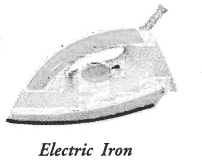2. Filament of an electric bulb is made of a thin wire of tungsten. The melting point of filament is high i.e., about 3380 °C. The filament of the bulb is enclosed in a glass envelope fixed over an insulated support as shown in figure 28. The glass envelope of electric bulb is filled with inactive gases like nitrogen and argon to increase the life of the tungsten filament.
Since resistance of thin filament is very high, so a large heat is produced as the electric current flows through the filament. Due to this large amount of heat produced, filament of the bulb becomes white hot. Hence, the filament of the bulb emits light and heat.3. Electric fuse is a safety device connected in series with the electric circuit. Electric fuse is a wire made of a material whose melting point is very low. Examples of the materials of making fuse wire are copper or tin-lead alloy. When large current flows through a circuit and hence through a fuse wire, a large amount of heat is produced. Due to this large amount of heat, the fuse wire melts and the circuit is broken so that current stops flowing in the circuit. This saves the electric circuit from burning due to the passage of large current through it.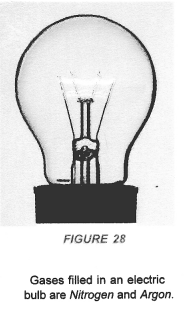Hope given Previous Year Question Papers for CBSE Class 10 Science Chapter 12 Electricity are helpful to complete your science homework.

If you have any doubts, please comment below. Learn Insta try to provide online science tutoring for you.